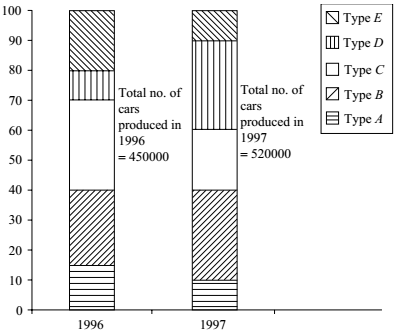## Introduction to Data Interpretation

#### Data Interpretation

Direction: Study the following graph carefully and then answer the questions based on it. The percentage of five different types of cars produced by a company during two years is given below.1. What was the difference in the production of C type cars between 1996 and 1997?

1. Production of C type cars in 1996 = (70 − 40)% of 450000
Production of C type cars in 1996 = 30% of 450000 = 135000

##### Correct Option: A

Production of C type cars in 1996 = (70 − 40)% of 450000
Production of C type cars in 1996 = 30% of 450000 = 135000
Production of C type cars in 1997 = (65 − 40)% of 520000
Production of C type cars in 1997 = 25% of 520000 = 130000
∴ Required difference = 5000.

1. If 85% of E type cars produced during 1996 and 1997 are being sold by the company, then how many E type cars are left unsold by the company?

1. Production of E type cars in 1996 = (100 − 80)% of 450000
Production of E type cars in 1996 = 20% of 450000 = 90000
And in 1997 = 10% of 520000 = 52000

##### Correct Option: E

Production of E type cars in 1996 = (100 − 80)% of 450000
Production of E type cars in 1996 = 20% of 450000 = 90000
And in 1997 = 10% of 520000 = 52000
∴ Total production = 90000 + 52000 = 142000.
∴ Required number of cars = 15% of 142000 = 21300

1. If the number of A type cars manufactured in 1997 was the same as that of 1996, what would have been its approximate percentage share in the total production of 1997?

1. Production of A type cars in 1997 = production of A type cars in 1996 (given) = (100 − 85) 5% of 450000 = 67500

##### Correct Option: B

Production of A type cars in 1997 = production of A type cars in 1996 (given) = (100 − 85) 5% of 450000 = 67500

 ∴ Required Percentage = 67500 X 100 ≈ 13 520000

1. In the case of which of the following types of cars was the percentage increase from 1996 to 1997 the maximum?

1. NA

##### Correct Option: C

Clearly, by visual inspection D is the desired option.

1. If the percentage production of B type cars in 1997 was the same as that of 1996, what would have been the number of cars produced in 1997?

1. Percentage production of B type cars in 1997 = that in 1996 (given)
= (40 − 15) 25% of 520000

##### Correct Option: C

Percentage production of B type cars in 1997 = that in 1996 (given)
= (40 − 15) 25% of 520000 = 130000.